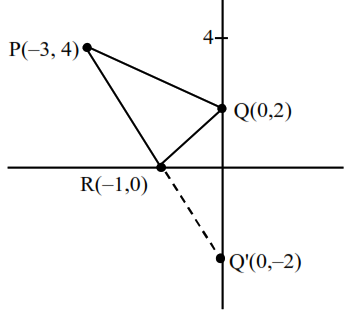# A man starts walking from`
Question:

A man starts walking from the point $\mathrm{P}(-3,4)$, touches the $\mathrm{x}$-axis at $\mathrm{R}$, and then turns to reach at the point $Q(0,2)$. The man is walking at a constant speed. If the man reaches the point $Q$ in the minimum time, then $50\left((\mathrm{PR})^{2}+(\mathrm{RQ})^{2}\right)$ is equal to

Solution:$50\left(\mathrm{PR}^{2}+\mathrm{RQ}^{2}\right)$

$50(20+5)$

$50(25)$

$=1250$